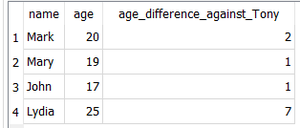Open in App
Not now

# ABS() and AVG() function in SQLite

• Last Updated : 08 Oct, 2021

1. ABS() Function :
ABS(X) is a math function used to get the absolute value of a number.

Syntax –

`ABS(X)`

The ABS(X) function takes a numeric argument X and returns its magnitude.

`SELECT ABS(-6);`

Output –

`6`

If the argument X is a blob or string, the function gives 0.0 as the return value

`SELECT ABS('gfg');`

Output –

`0.0`

If the argument X is NULL the function returns NULL.

`SELECT ABS(NULL);`

Output –

`NULL`

2. AVG() Function :
AVG(X) is an aggregate function used to calculate the average on a set of rows.

Syntax –

`AVG(X)`

The function yields a floating point value equal to the average of all NON-NULL values in the argument group X.

`SELECT AVG(1,2,3,4,5,6,7)`

Output –

`4.0`

If there is a NULL value passed to the AVG() function, only  the non-NULL values are used in calculating the average.

`SELECT AVG(10,20,30,NULL);`

Output –

`20.0`

Strings and blobs present in the argument X are evaluated as 0.

`SELECT AVG(10,20,'word',30,);`

Output –

`20.0`

The function returns a NULL if all the input values are NULL.

`SELECT AVG(NULL,NULL,NULL);`

Output –

`NULL`

Now, we are going to use a real database example. First, let’s create a table called students with columns name and age and insert some rows!

```--create a table
CREATE TABLE students(
id INTEGER PRIMARY KEY AUTOINCREMENT,
name VARCHAR(255),
age INT);

--insert multiple rows
INSERT INTO students(name,age)
VALUES
('Mark',20),
('Mary',19),
('John',17),
('Lydia',25);```

We’ll  then write a SELECT statement that gives us the average age for all the students using the AVG() function

`SELECT AVG(age) FROM students;`

Output –

`20.25`

Next, we will create a SELECT statement that returns the age difference between each student and a new student Tony who is aged 18 years.

```SELECT
name,
age,
ABS(age-18) AS age_difference_against_Tony
FROM students;```

Output –Output of a SELECT statement using ABS() function

My Personal Notes arrow_drop_up